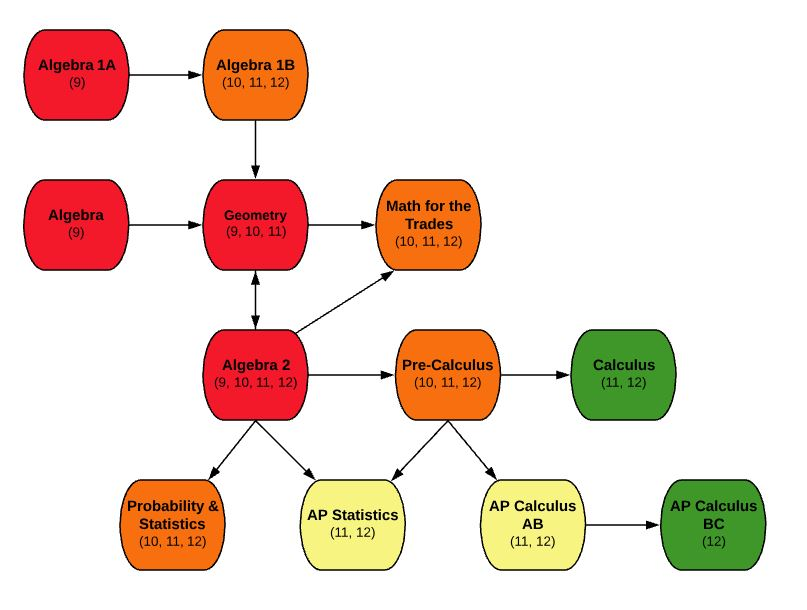EDIT MAIN

# MA710 AP StatisticsCourse: MA710 AP Statistics

Subject Area: Advanced Placement, Articulated Credits, Mathematics

Credits: 1

Course Information:

This is a year-long course that requires selection of both semesters: S1 and S2. Accelerated College Advanced Program AP Statistics will introduce students to the major concepts and tools for collecting, analyzing, and drawing conclusions from data. The main topics will include exploring data, planning a study, anticipating patterns, and statistical inference. All topics will be studied extensively with the use of graphing calculators and computer software. Students are expected to take the Advanced Placement exam in May. Ample time will be provided to review exam topics prior to the AP Exam. A TI-83 or TI-84 graphing calculator is required for this course.

## Outcomes:

• Construct and interpret distributions with graphs, charts, and tables
• Describe and compare distributions
• Calculate numerical summaries by hand and using technology
• Describe and interpret locations in a distribution (z-scores, percentiles, etc.)
• Perform Normal distribution calculations including assessing normality
• Construct and interpret scatterplots
• Measure, calculate and interpret correlation and a least square regression line
• Interpret computer output Describe different sampling methods (Stratified, Cluster, etc.)
• Conduct simple random samples (using a random number table, and technology)
• Design comparative experiments (completely randomized, randomized block, and matched pairs designs)
• Use rules of probability to construct venn diagrams, two-way tables and determine probabilities
• Apply the concept of discrete random variables to many different statistical settings
• Calculate the expected values of a discrete random variable
• Describe continuous random variables
• Compute probabilities involving binomial and geometric random variables
• Define and distinguish between population distribution, sampling distribution, and the distribution of sample data
• Estimate a population proportion and mean using confidence intervals
• Interpret confidence intervals in context
• Conduct significance tests with populations proportions and means
• Conduct chi-square goodness of fit tests

Prerequisites:

Minimum grade of C- in Pre-Calculus or Algebra II

Corequisites:

If you take this course, you must also take MA710-S2 – AP Statistics S2

Notes: A TI-83 or TI-84 graphing calculator is required for this course.